Do you know the FOIL system?

# Algebra Intermediate - Factoring Difference of Squares

This Math quiz is called 'Algebra Intermediate - Factoring Difference of Squares' and it has been written by teachers to help you if you are studying the subject at middle school. Playing educational quizzes is a fabulous way to learn if you are in the 6th, 7th or 8th grade - aged 11 to 14.

It costs only \$12.50 per month to play this quiz and over 3,500 others that help you with your school work. You can subscribe on the page at Join Us

As you are aware, when “factoring” in math, it means that the problem you will be working on will involve multiplication. It can also involve other mathematical methods such as adding and subtracting. In this quiz, you will be factoring difference of squares. What does that mean?

First off, when factoring differences of squares you will be working with two binomial factors and then you will be using what is called the FOIL system. You more than likely learned the FOIL system in pre-algebra but as a quick refresher, the FOIL system refers to multiplying two factors that are surrounded by parentheses.

You multiply the first term in each factor as your first step, then the first term in the first factor (which is also considered an “outer” term) with the “outer” term (or second term) in the second factor. The third step is to multiply the two inner terms, (i.e., the second term of the first factor and the first term of the second factor) and, finally, you multiply the last two terms of each factor. Let’s look at the example below.

Go straight to Quiz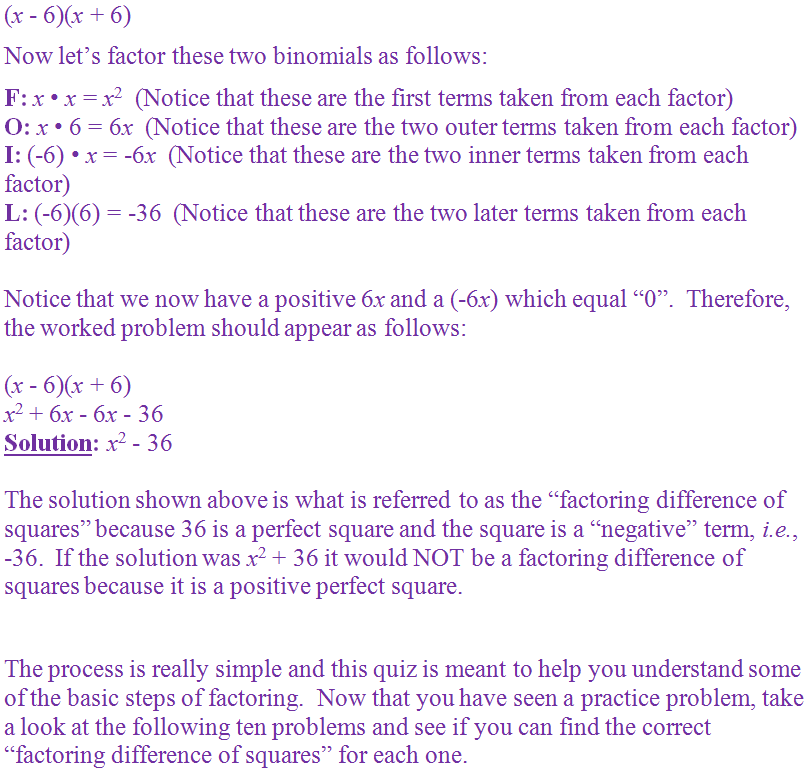1.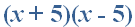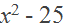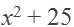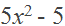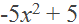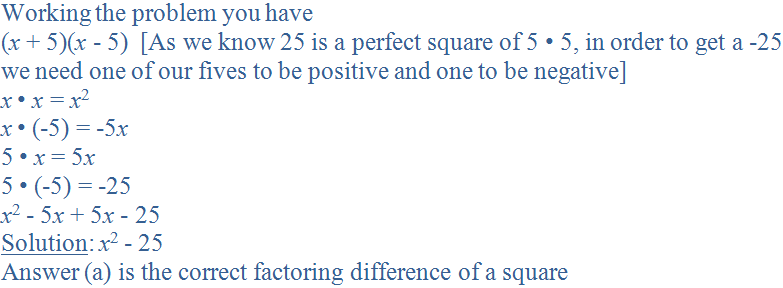2.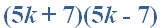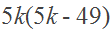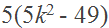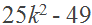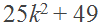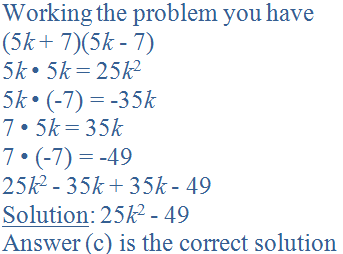3.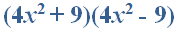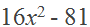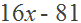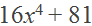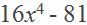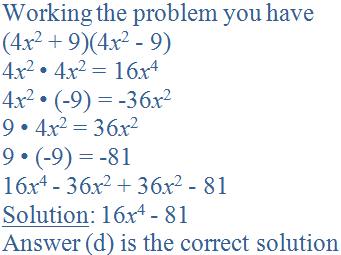4.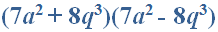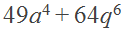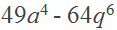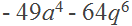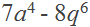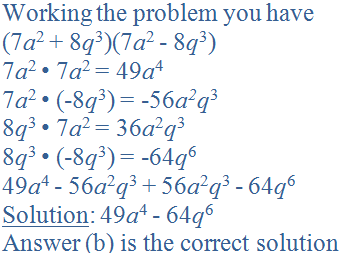5.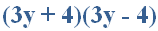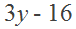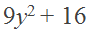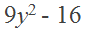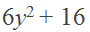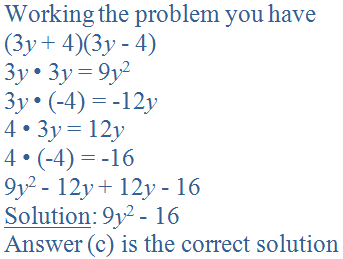6.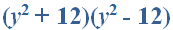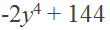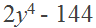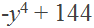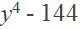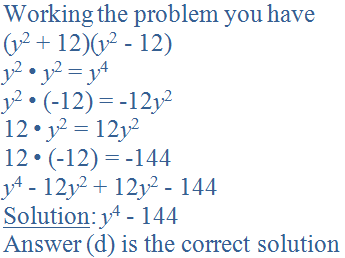7.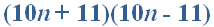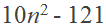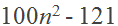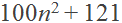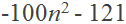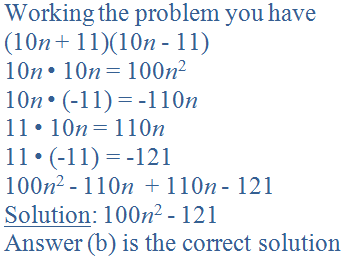8.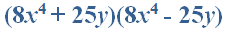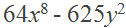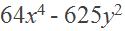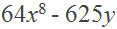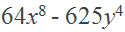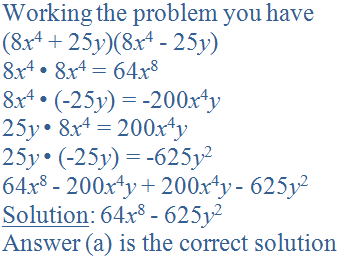9.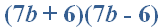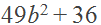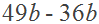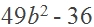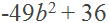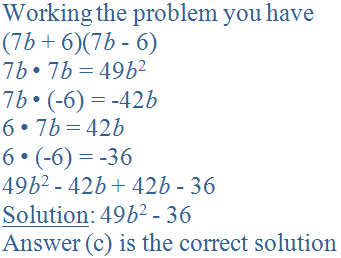10.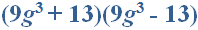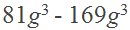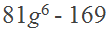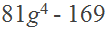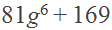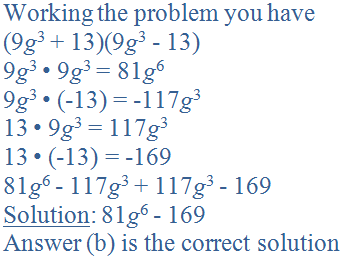Author:  Christine G. Broome

We use cookies to make your experience of our website better.

To comply with the new e-Privacy directive, we need to ask for your consent -